JEE  >  Test: Relations & Functions- 2

# Test: Relations & Functions- 2

Test Description

## 25 Questions MCQ Test Mathematics (Maths) Class 12 | Test: Relations & Functions- 2

Test: Relations & Functions- 2 for JEE 2023 is part of Mathematics (Maths) Class 12 preparation. The Test: Relations & Functions- 2 questions and answers have been prepared according to the JEE exam syllabus.The Test: Relations & Functions- 2 MCQs are made for JEE 2023 Exam. Find important definitions, questions, notes, meanings, examples, exercises, MCQs and online tests for Test: Relations & Functions- 2 below.
Solutions of Test: Relations & Functions- 2 questions in English are available as part of our Mathematics (Maths) Class 12 for JEE & Test: Relations & Functions- 2 solutions in Hindi for Mathematics (Maths) Class 12 course. Download more important topics, notes, lectures and mock test series for JEE Exam by signing up for free. Attempt Test: Relations & Functions- 2 | 25 questions in 25 minutes | Mock test for JEE preparation | Free important questions MCQ to study Mathematics (Maths) Class 12 for JEE Exam | Download free PDF with solutions
 1 Crore+ students have signed up on EduRev. Have you?
Test: Relations & Functions- 2 - Question 1

### R = {(1, 1), (2, 2), (1, 2), (2, 1), (2, 3)} be a relation on A = {1, 2, 3}, then R is

Detailed Solution for Test: Relations & Functions- 2 - Question 1

A relation R on a non empty set A is said to be reflexive if fx Rx for all x ∈ R, Therefore , R is not reflexive.
A relation R on a non empty set A is said to be symmetric if fx Ry ⇔ yRx, for all x, y ∈ R Therefore, R is not symmetric.
A relation R on a non empty set A is said to be antisymmetric if fx Ry and y Rx ⇒ x = y, for all x, y ∈ R. Therefore, R is not antisymmetric.

Test: Relations & Functions- 2 - Question 2

### If R is a relation from a set A to a set B and S is a relation from B to C, then the relation S ∘ R.

Detailed Solution for Test: Relations & Functions- 2 - Question 2

If R is a relation from A→B and S is a relation fromB→C, then S ∘ R is a composite function from A to C.

Test: Relations & Functions- 2 - Question 3

### Let A be the set of all students of a boys school. The relation R in A given by R = {(a, b) : a is sister of b} is

Detailed Solution for Test: Relations & Functions- 2 - Question 3

Because, the relation is defined over the set A which is the set of all students of a boys school.

Test: Relations & Functions- 2 - Question 4

The domain of definition of the function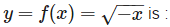Detailed Solution for Test: Relations & Functions- 2 - Question 4

y is defined  If -x ≥ 0, i.e.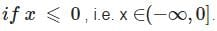Test: Relations & Functions- 2 - Question 5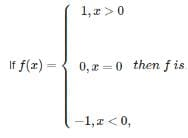Detailed Solution for Test: Relations & Functions- 2 - Question 5

By definition, The Signum function =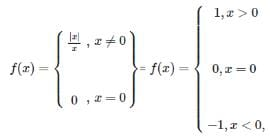Test: Relations & Functions- 2 - Question 6

Let A = {1, 2, 3}. Which of the following is not an equivalence relation on A ?

Detailed Solution for Test: Relations & Functions- 2 - Question 6

A relation R on a non empty set A is said to be reflexive iff xRx for all x ∈ R . A relation R on a non empty set A is said to be symmetric iff xRy ⇔ yRx, for all x , y ∈ R .
A relation R on a non empty set A is said to be transitive iff xRy and yRz ⇒ xRz, for all x ∈ R. An equivalence relation satisfies all these three properties.
None of the given relations satisfies all three properties of equivalence relation.

Test: Relations & Functions- 2 - Question 7

The binary operation * defined on the set of integers as a∗b = |a−b|-1 is:

Detailed Solution for Test: Relations & Functions- 2 - Question 7

Here * is commutative as b*a = |b−a|−1 = |a−b|−1 = a∗b.
Because ,|−x| = |x| for all x ∈ R.

Test: Relations & Functions- 2 - Question 8

Let T be the set of all triangles in a plane with R a relation in T given by R = {(T1, T2) : T1 is congruent to T2}. Then R is

Detailed Solution for Test: Relations & Functions- 2 - Question 8

Let T be the set of all triangles in a plane with R a relation in T given by R = {(T1, T2): T1 is congruent to T2}. (T1T2) ∈ R iff T1 is congruent to T2
Reflexivity :T1≅T1 ⇒ (T1T1) ∈ R . Symmetry :(T1, T2)∈ R ⇒T1≅T2 ⇒ T2≅T1 ⇒ (T2, T1) ∈ R
Transitivity :(T1,T1) ∈ R and (T2, T3) ∈ R ⇒ T1≅T2 and T2≅T3 ⇒ T1≅T3 ⇒ (T1, T3) ∈ R .
Therefore, R is an equivalence relation on T.

Test: Relations & Functions- 2 - Question 9

The range of the function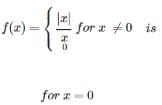Detailed Solution for Test: Relations & Functions- 2 - Question 9

The given function is defined as Signum function. i.e.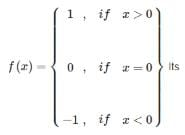domain is R and Range is {-1 , 0 , 1}.

Test: Relations & Functions- 2 - Question 10

The function f (x) = x2+ sin x is

Detailed Solution for Test: Relations & Functions- 2 - Question 10

For even function : f(-x) = f(x) , therefore, f(- x) = (− x)2+ sin (− x)
= x2−sin x ≠ f(x).
For an odd function : f(-x) = - f(x) , therefore, f(-x) = (− x)2+ sin (− x)
= x2− sin x
≠ − f(x).
Therefore f(x) is neither even nor odd.

Test: Relations & Functions- 2 - Question 11

If A is a finite set containing n distinct elements, then the number of relations on A is equal to

Detailed Solution for Test: Relations & Functions- 2 - Question 11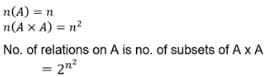Test: Relations & Functions- 2 - Question 12

R is a relation from { 11, 12, 13} to {8, 10, 12} defined by y = x – 3. The relation R−1

Detailed Solution for Test: Relations & Functions- 2 - Question 12

R is a relation from {11, 12, 13} to {8, 10, 12} defined by y = x – 3. The relation is given by x = y + 3,from{8, 10, 12} to {11, 12, 13} ⇒ relation = {(8,11),(10,13)}.

Test: Relations & Functions- 2 - Question 13

If A = {(1, 2, 3}, then the relation R = {(2, 3)} in A is

Detailed Solution for Test: Relations & Functions- 2 - Question 13

A = {1,2,3}
B = {(2,3)} is not reflexive or symmetric on A but it is transitive
∵ if (a,b) exists but (b,c) does not exist then (a,c) does not need to exist and the relation is still transitive.

Test: Relations & Functions- 2 - Question 14

The range of the function f(x) = [sin x] is

Detailed Solution for Test: Relations & Functions- 2 - Question 14

The only possible integral values of sin x are {-1 ,0, 1}.

Test: Relations & Functions- 2 - Question 15

The period of the function f(x) = sin2x + tan x is

Detailed Solution for Test: Relations & Functions- 2 - Question 15

We have the function f(x) = sin2x + tan x, therefore, f(π+x)
= {sin2(π+x)+tan(π+x)}
= {sin2x+tanx} = f(x).
This implies that period of the function f(x) is π.

Test: Relations & Functions- 2 - Question 16

Let A = {1, 2, 3}, then the domain of the relation R = {(1, 1), (2, 3), (2, 1)} defined on A is

Detailed Solution for Test: Relations & Functions- 2 - Question 16

Since the domain is represented by the x- co ordinate of the ordered pair (x , y).Therefore, domain of the given relation is {1, 2}.

Test: Relations & Functions- 2 - Question 17

Given the relation R = {(1, 2), (2, 3)} on eht set {1, 2, 3}, the minimum number of ordered pairs which when added to R make it an equivalence

Detailed Solution for Test: Relations & Functions- 2 - Question 17

To make the relation an equivalence relation , the following ordered pairs are required (1,1),(2,2),(3,3)(2,1)(3,2)(1,3),(3,1)

Test: Relations & Functions- 2 - Question 18

Let R = {(1, 3), (4, 2), (2, 4), (2, 3), (3, 1)} be a relations on the set A = {1, 2, 3, 4}. the relation R is

Detailed Solution for Test: Relations & Functions- 2 - Question 18

Given, R = {(1, 3), (4, 2), (2, 4), (2, 3), (3, 1)} be a relation on the set A = {1, 2, 3, 4}.
(a) Since, (1, 1), (2, 2), (3, 3), (4, 4) ∉ R. So, R is not reflexive.
(b) Since, (1, 3) ∈ R and (3, 1) ∈ R but (1, 1) ∉ R. So, R is not transitive.
(c) Since, (2, 3) ∈ R but (3,2) ∉ R. So, B is not symmetric.
(d) Since, (2, 4) ∈ R and (2, 3) ∈ R. So, R is not a function.

Test: Relations & Functions- 2 - Question 19

The relation R in the set Z of integers given by R = {(a, b): 2 divides a – b}(a, b) where a, b ∈ Z is

Detailed Solution for Test: Relations & Functions- 2 - Question 19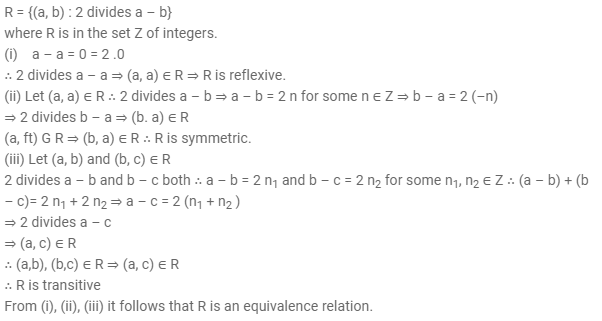Test: Relations & Functions- 2 - Question 20

The range of the function f(x) = x – [x] is

Detailed Solution for Test: Relations & Functions- 2 - Question 20

Since [x] ≤ x, therefore , x – [x] ≥ 0. Also, x – [x] <1,∴0⩽x−[x]<1. Therefore ,Rf = [0,1).

Test: Relations & Functions- 2 - Question 21

Let A contain n distinct numbers. How many bijections from A to A can be defined?

Detailed Solution for Test: Relations & Functions- 2 - Question 21

A bijection from A to A is infact an arrangement of its n elements, taken all at a time, which can be done in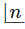ways. Hence, the number of bijections from A to A isTest: Relations & Functions- 2 - Question 22

Number of relations that can be defined on the set A = {a, b, c, d} is

Detailed Solution for Test: Relations & Functions- 2 - Question 22

No. of elements in the set A = 4. Therefore , the no. of elements in A × A = 4 × 4 = 16. As, the no. of relations in A × A = no. of subsets of A × A = 216

Test: Relations & Functions- 2 - Question 23

A relation R in a set A is called universal relation, if

Detailed Solution for Test: Relations & Functions- 2 - Question 23

The relation R = A x A is called Universal relation.

Test: Relations & Functions- 2 - Question 24

Let R be the relation defined in the set A = {1, 2, 3, 4, 5, 6, 7} by R = {(a, b) : both a and b are either odd or even}. Then R is

Detailed Solution for Test: Relations & Functions- 2 - Question 24

Consider any a ,b , c ∈A .

1) Since both a and a must be either even or odd, so (a , a) ∈R ⇒ R is reflexive.

2) Let (a ,b) ∈R ⇒ both a and b must be either even or odd, ⇒ both b and a must be either even or odd, ⇒ (b ,a) ∈R. Thus , (a ,b) ∈R ⇒ (b ,a) ∈R ⇒ R is symmetric.

3) Let (a ,b) ∈R and (b ,c) ∈R ⇒ both a and b must be either even or odd, also ,both b and c must be either even or odd, ⇒ all elements a, b and c must be either even or odd, ⇒ (a ,c) ∈R . Thus, (a ,b) ∈R ⇒ (b,c) ∈R ⇒ (a ,c) ∈R ⇒ R is transitive.

Test: Relations & Functions- 2 - Question 25

If f(x) = x – x2, then f(a + 1) – f(a – 1) , a ∈ R is :

Detailed Solution for Test: Relations & Functions- 2 - Question 25

f(a+1)−f(a−1)
=(a+1)−(a+1)2−{(a−1)−(a−1)2} = 2−4a(a−1)2} = 2−4a

## Mathematics (Maths) Class 12

209 videos|218 docs|139 tests
Information about Test: Relations & Functions- 2 Page
In this test you can find the Exam questions for Test: Relations & Functions- 2 solved & explained in the simplest way possible. Besides giving Questions and answers for Test: Relations & Functions- 2, EduRev gives you an ample number of Online tests for practice

## Mathematics (Maths) Class 12

209 videos|218 docs|139 tests(Scan QR code)